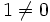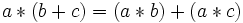# Left quasifield

## Definition

A left quasifield is a set$Q$ equipped with:

• A (infix) binary operation$+$, called the addition or additive operation.
• A unary operation$-$, called the additive inverse.
• A constant$0$, called zero.
• A (infix) binary operation$*$, called the multiplication.
• A constant$1$ such that$1 \ne 0$.

Such that:

•$(Q,+,0,-)$ is a group.
•$(Q \setminus \{ 0 \},*,1,)$ is a Moufang loop with multiplication$*$ and identity element$1$.
•$\! a * (b + c) = (a * b) + (a * c)$ (i.e., we have the left distributive law).
•$\! a * x = (b * x) + c$ has exactly one solution in$x$ for any fixed$a,b,c \in Q$ with$a \ne b$.